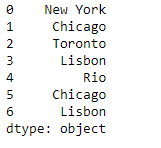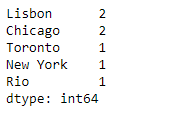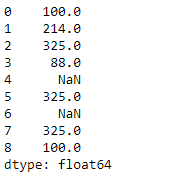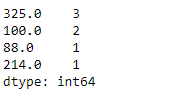Open in App
Not now

# Python | Pandas Series.value_counts()

• Last Updated : 29 Jan, 2019

Python is a great language for doing data analysis, primarily because of the fantastic ecosystem of data-centric python packages. Pandas is one of those packages and makes importing and analyzing data much easier.

Pandas series is a One-dimensional ndarray with axis labels. The labels need not be unique but must be a hashable type. The object supports both integer- and label-based indexing and provides a host of methods for performing operations involving the index.

Pandas` Series.value_counts()` function return a Series containing counts of unique values. The resulting object will be in descending order so that the first element is the most frequently-occurring element. Excludes NA values by default.

Syntax: Series.value_counts(normalize=False, sort=True, ascending=False, bins=None, dropna=True)

Parameter :
normalize : If True then the object returned will contain the relative frequencies of the unique values.
sort : Sort by values.
ascending : Sort in ascending order.
bins : Rather than count values, group them into half-open bins, a convenience for pd.cut, only works with numeric data.
dropna : Don’t include counts of NaN.

Returns : counts : Series

Example #1: Use `Series.value_counts()` function to find the unique value counts of each element in the given Series object.

 `# importing pandas as pd``import` `pandas as pd`` ` `# Creating the Series``sr ``=` `pd.Series([``'New York'``, ``'Chicago'``, ``'Toronto'``, ``'Lisbon'``, ``'Rio'``, ``'Chicago'``, ``'Lisbon'``])`` ` `# Print the series``print``(sr)`

Output :Now we will use `Series.value_counts()` function to find the values counts of each unique value in the given Series object.

 `# find the value counts``sr.value_counts()`

Output :As we can see in the output, the `Series.value_counts()` function has returned the value counts of each unique value in the given Series object.

Example #2: Use `Series.value_counts()` function to find the unique value counts of each element in the given Series object.

 `# importing pandas as pd``import` `pandas as pd`` ` `# Creating the Series``sr ``=` `pd.Series([``100``, ``214``, ``325``, ``88``, ``None``, ``325``, ``None``, ``325``, ``100``])`` ` `# Print the series``print``(sr)`

Output :Now we will use `Series.value_counts()` function to find the values counts of each unique value in the given Series object.

 `# find the value counts``sr.value_counts()`

Output :As we can see in the output, the `Series.value_counts()` function has returned the value counts of each unique value in the given Series object.

My Personal Notes arrow_drop_up